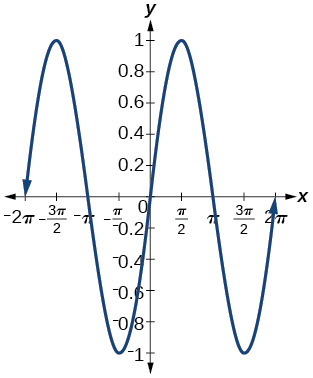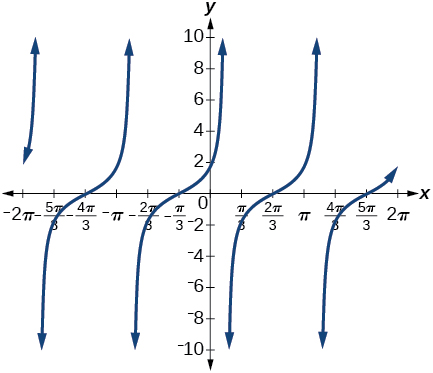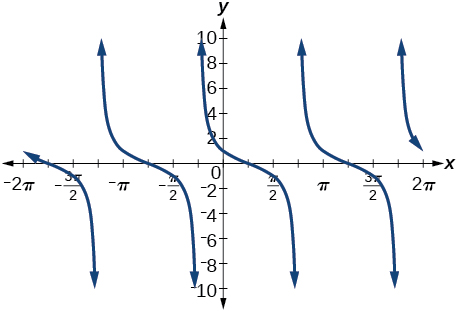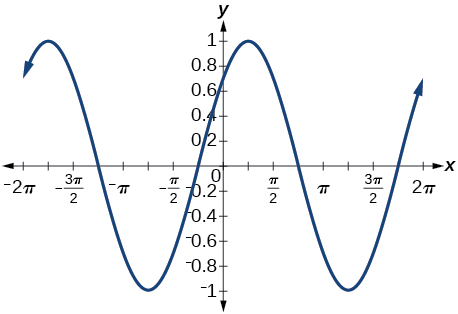# 9.2 Sum and difference identities  (Page 6/6)

 Page 6 / 6

## Verbal

Explain the basis for the cofunction identities and when they apply.

The cofunction identities apply to complementary angles. Viewing the two acute angles of a right triangle, if one of those angles measures $\text{\hspace{0.17em}}x,$ the second angle measures $\text{\hspace{0.17em}}\frac{\pi }{2}-x.\text{\hspace{0.17em}}$ Then $\text{\hspace{0.17em}}\mathrm{sin}x=\mathrm{cos}\left(\frac{\pi }{2}-x\right).\text{\hspace{0.17em}}$ The same holds for the other cofunction identities. The key is that the angles are complementary.

Is there only one way to evaluate $\text{\hspace{0.17em}}\mathrm{cos}\left(\frac{5\pi }{4}\right)?\text{\hspace{0.17em}}$ Explain how to set up the solution in two different ways, and then compute to make sure they give the same answer.

Explain to someone who has forgotten the even-odd properties of sinusoidal functions how the addition and subtraction formulas can determine this characteristic for $\text{\hspace{0.17em}}f\left(x\right)=\mathrm{sin}\left(x\right)\text{\hspace{0.17em}}$ and $\text{\hspace{0.17em}}g\left(x\right)=\mathrm{cos}\left(x\right).\text{\hspace{0.17em}}$ (Hint: $\text{\hspace{0.17em}}0-x=-x$ )

$\mathrm{sin}\left(-x\right)=-\mathrm{sin}x,$ so $\text{\hspace{0.17em}}\mathrm{sin}x\text{\hspace{0.17em}}$ is odd. $\text{\hspace{0.17em}}\mathrm{cos}\left(-x\right)=\mathrm{cos}\left(0-x\right)=\mathrm{cos}x,$ so $\text{\hspace{0.17em}}\mathrm{cos}x\text{\hspace{0.17em}}$ is even.

## Algebraic

For the following exercises, find the exact value.

$\mathrm{cos}\left(\frac{7\pi }{12}\right)$

$\mathrm{cos}\left(\frac{\pi }{12}\right)$

$\frac{\sqrt{2}+\sqrt{6}}{4}$

$\mathrm{sin}\left(\frac{5\pi }{12}\right)$

$\mathrm{sin}\left(\frac{11\pi }{12}\right)$

$\frac{\sqrt{6}-\sqrt{2}}{4}$

$\mathrm{tan}\left(-\frac{\pi }{12}\right)$

$\mathrm{tan}\left(\frac{19\pi }{12}\right)$

$-2-\sqrt{3}$

For the following exercises, rewrite in terms of $\text{\hspace{0.17em}}\mathrm{sin}\text{\hspace{0.17em}}x\text{\hspace{0.17em}}$ and $\text{\hspace{0.17em}}\mathrm{cos}\text{\hspace{0.17em}}x.$

$\mathrm{sin}\left(x+\frac{11\pi }{6}\right)$

$\mathrm{sin}\left(x-\frac{3\pi }{4}\right)$

$-\frac{\sqrt{2}}{2}\mathrm{sin}x-\frac{\sqrt{2}}{2}\mathrm{cos}x$

$\mathrm{cos}\left(x-\frac{5\pi }{6}\right)$

$\mathrm{cos}\left(x+\frac{2\pi }{3}\right)$

$-\frac{1}{2}\mathrm{cos}x-\frac{\sqrt{3}}{2}\mathrm{sin}x$

For the following exercises, simplify the given expression.

$\mathrm{csc}\left(\frac{\pi }{2}-t\right)$

$\mathrm{sec}\left(\frac{\pi }{2}-\theta \right)$

$\mathrm{csc}\theta$

$\mathrm{cot}\left(\frac{\pi }{2}-x\right)$

$\mathrm{tan}\left(\frac{\pi }{2}-x\right)$

$\mathrm{cot}x$

$\mathrm{sin}\left(2x\right)\text{\hspace{0.17em}}\mathrm{cos}\left(5x\right)-\mathrm{sin}\left(5x\right)\text{\hspace{0.17em}}\mathrm{cos}\left(2x\right)$

$\frac{\mathrm{tan}\left(\frac{3}{2}x\right)-\mathrm{tan}\left(\frac{7}{5}x\right)}{1+\mathrm{tan}\left(\frac{3}{2}x\right)\mathrm{tan}\left(\frac{7}{5}x\right)}$

$\mathrm{tan}\left(\frac{x}{10}\right)$

For the following exercises, find the requested information.

Given that $\text{\hspace{0.17em}}\mathrm{sin}\text{\hspace{0.17em}}a=\frac{2}{3}\text{\hspace{0.17em}}$ and $\text{\hspace{0.17em}}\mathrm{cos}\text{\hspace{0.17em}}b=-\frac{1}{4},$ with $\text{\hspace{0.17em}}a\text{\hspace{0.17em}}$ and $\text{\hspace{0.17em}}b\text{\hspace{0.17em}}$ both in the interval $\text{\hspace{0.17em}}\left[\frac{\pi }{2},\pi \right),$ find $\text{\hspace{0.17em}}\mathrm{sin}\left(a+b\right)\text{\hspace{0.17em}}$ and $\text{\hspace{0.17em}}\mathrm{cos}\left(a-b\right).$

Given that $\text{\hspace{0.17em}}\mathrm{sin}\text{\hspace{0.17em}}a=\frac{4}{5},$ and $\text{\hspace{0.17em}}\mathrm{cos}\text{\hspace{0.17em}}b=\frac{1}{3},$ with $\text{\hspace{0.17em}}a\text{\hspace{0.17em}}$ and $\text{\hspace{0.17em}}b\text{\hspace{0.17em}}$ both in the interval $\text{\hspace{0.17em}}\left[0,\frac{\pi }{2}\right),$ find $\text{\hspace{0.17em}}\mathrm{sin}\left(a-b\right)\text{\hspace{0.17em}}$ and $\text{\hspace{0.17em}}\mathrm{cos}\left(a+b\right).$

$\begin{array}{ccccc}\hfill \mathrm{sin}\left(a-b\right)& =& \left(\frac{4}{5}\right)\left(\frac{1}{3}\right)-\left(\frac{3}{5}\right)\left(\frac{2\sqrt{2}}{3}\right)\hfill & =& \frac{4-6\sqrt{2}}{15}\hfill \\ \hfill \mathrm{cos}\left(a+b\right)& =& \left(\frac{3}{5}\right)\left(\frac{1}{3}\right)-\left(\frac{4}{5}\right)\left(\frac{2\sqrt{2}}{3}\right)\hfill & =& \frac{3-8\sqrt{2}}{15}\hfill \end{array}$

For the following exercises, find the exact value of each expression.

$\mathrm{sin}\left({\mathrm{cos}}^{-1}\left(0\right)-{\mathrm{cos}}^{-1}\left(\frac{1}{2}\right)\right)$

$\mathrm{cos}\left({\mathrm{cos}}^{-1}\left(\frac{\sqrt{2}}{2}\right)+{\mathrm{sin}}^{-1}\left(\frac{\sqrt{3}}{2}\right)\right)$

$\frac{\sqrt{2}-\sqrt{6}}{4}$

$\mathrm{tan}\left({\mathrm{sin}}^{-1}\left(\frac{1}{2}\right)-{\mathrm{cos}}^{-1}\left(\frac{1}{2}\right)\right)$

## Graphical

For the following exercises, simplify the expression, and then graph both expressions as functions to verify the graphs are identical. Confirm your answer using a graphing calculator.

$\mathrm{cos}\left(\frac{\pi }{2}-x\right)$

$\mathrm{sin}x$$\mathrm{sin}\left(\pi -x\right)$

$\mathrm{tan}\left(\frac{\pi }{3}+x\right)$

$\mathrm{cot}\left(\frac{\pi }{6}-x\right)$$\mathrm{sin}\left(\frac{\pi }{3}+x\right)$

$\mathrm{tan}\left(\frac{\pi }{4}-x\right)$

$\mathrm{cot}\left(\frac{\pi }{4}+x\right)$$\mathrm{cos}\left(\frac{7\pi }{6}+x\right)$

$\mathrm{sin}\left(\frac{\pi }{4}+x\right)$

$\frac{\mathrm{sin}x}{\sqrt{2}}+\frac{\mathrm{cos}x}{\sqrt{2}}$$\mathrm{cos}\left(\frac{5\pi }{4}+x\right)$

For the following exercises, use a graph to determine whether the functions are the same or different. If they are the same, show why. If they are different, replace the second function with one that is identical to the first. (Hint: think $\text{\hspace{0.17em}}2x=x+x.$ )

$f\left(x\right)=\mathrm{sin}\left(4x\right)-\mathrm{sin}\left(3x\right)\mathrm{cos}\text{\hspace{0.17em}}x,g\left(x\right)=\mathrm{sin}\text{\hspace{0.17em}}x\text{\hspace{0.17em}}\mathrm{cos}\left(3x\right)$

They are the same.

$f\left(x\right)=\mathrm{cos}\left(4x\right)+\mathrm{sin}\text{\hspace{0.17em}}x\text{\hspace{0.17em}}\mathrm{sin}\left(3x\right),g\left(x\right)=-\mathrm{cos}\text{\hspace{0.17em}}x\text{\hspace{0.17em}}\mathrm{cos}\left(3x\right)$

$f\left(x\right)=\mathrm{sin}\left(3x\right)\mathrm{cos}\left(6x\right),g\left(x\right)=-\mathrm{sin}\left(3x\right)\mathrm{cos}\left(6x\right)$

They are the different, try $\text{\hspace{0.17em}}g\left(x\right)=\mathrm{sin}\left(9x\right)-\mathrm{cos}\left(3x\right)\mathrm{sin}\left(6x\right).$

$f\left(x\right)=\mathrm{sin}\left(4x\right),g\left(x\right)=\mathrm{sin}\left(5x\right)\mathrm{cos}\text{\hspace{0.17em}}x-\mathrm{cos}\left(5x\right)\mathrm{sin}\text{\hspace{0.17em}}x$

$f\left(x\right)=\mathrm{sin}\left(2x\right),g\left(x\right)=2\text{\hspace{0.17em}}\mathrm{sin}\text{\hspace{0.17em}}x\text{\hspace{0.17em}}\mathrm{cos}\text{\hspace{0.17em}}x$

They are the same.

$f\left(\theta \right)=\mathrm{cos}\left(2\theta \right),g\left(\theta \right)={\mathrm{cos}}^{2}\theta -{\mathrm{sin}}^{2}\theta$

$f\left(\theta \right)=\mathrm{tan}\left(2\theta \right),g\left(\theta \right)=\frac{\mathrm{tan}\text{\hspace{0.17em}}\theta }{1+{\mathrm{tan}}^{2}\theta }$

They are the different, try $\text{\hspace{0.17em}}g\left(\theta \right)=\frac{2\text{\hspace{0.17em}}\mathrm{tan}\theta }{1-{\mathrm{tan}}^{2}\theta }.$

$f\left(x\right)=\mathrm{sin}\left(3x\right)\mathrm{sin}\text{\hspace{0.17em}}x,g\left(x\right)={\mathrm{sin}}^{2}\left(2x\right){\mathrm{cos}}^{2}x-{\mathrm{cos}}^{2}\left(2x\right){\mathrm{sin}}^{2}x$

$f\left(x\right)=\mathrm{tan}\left(-x\right),g\left(x\right)=\frac{\mathrm{tan}\text{\hspace{0.17em}}x-\mathrm{tan}\left(2x\right)}{1-\mathrm{tan}\text{\hspace{0.17em}}x\text{\hspace{0.17em}}\mathrm{tan}\left(2x\right)}$

They are different, try $\text{\hspace{0.17em}}g\left(x\right)=\frac{\mathrm{tan}x-\mathrm{tan}\left(2x\right)}{1+\mathrm{tan}x\mathrm{tan}\left(2x\right)}.$

## Technology

For the following exercises, find the exact value algebraically, and then confirm the answer with a calculator to the fourth decimal point.

$\mathrm{sin}\left(75°\right)$

$\mathrm{sin}\left(195°\right)$

$\mathrm{cos}\left(165°\right)$

$\mathrm{cos}\left(345°\right)$

$\frac{1+\sqrt{3}}{2\sqrt{2}},$ or 0.9659

$\mathrm{tan}\left(-15°\right)$

## Extensions

For the following exercises, prove the identities provided.

$\mathrm{tan}\left(x+\frac{\pi }{4}\right)=\frac{\mathrm{tan}\text{\hspace{0.17em}}x+1}{1-\mathrm{tan}\text{\hspace{0.17em}}x}$

$\begin{array}{ccc}\hfill \mathrm{tan}\left(x+\frac{\pi }{4}\right)& =& \\ \hfill \frac{\mathrm{tan}x+\mathrm{tan}\left(\frac{\pi }{4}\right)}{1-\mathrm{tan}x\mathrm{tan}\left(\frac{\pi }{4}\right)}& =& \\ \hfill \frac{\mathrm{tan}x+1}{1-\mathrm{tan}x\left(1\right)}& =& \frac{\mathrm{tan}x+1}{1-\mathrm{tan}x}\hfill \end{array}$

$\frac{\mathrm{tan}\left(a+b\right)}{\mathrm{tan}\left(a-b\right)}=\frac{\mathrm{sin}\text{\hspace{0.17em}}a\text{\hspace{0.17em}}\mathrm{cos}\text{\hspace{0.17em}}a+\mathrm{sin}\text{\hspace{0.17em}}b\text{\hspace{0.17em}}\mathrm{cos}\text{\hspace{0.17em}}b}{\mathrm{sin}\text{\hspace{0.17em}}a\text{\hspace{0.17em}}\mathrm{cos}\text{\hspace{0.17em}}a-\mathrm{sin}\text{\hspace{0.17em}}b\text{\hspace{0.17em}}\mathrm{cos}\text{\hspace{0.17em}}b}$

$\frac{\mathrm{cos}\left(a+b\right)}{\mathrm{cos}\text{\hspace{0.17em}}a\text{\hspace{0.17em}}\mathrm{cos}\text{\hspace{0.17em}}b}=1-\mathrm{tan}\text{\hspace{0.17em}}a\text{\hspace{0.17em}}\mathrm{tan}\text{\hspace{0.17em}}b$

$\begin{array}{ccc}\hfill \frac{\mathrm{cos}\left(a+b\right)}{\mathrm{cos}a\mathrm{cos}b}& =& \\ \hfill \frac{\mathrm{cos}a\mathrm{cos}b}{\mathrm{cos}a\mathrm{cos}b}-\frac{\mathrm{sin}a\mathrm{sin}b}{\mathrm{cos}a\mathrm{cos}b}& =& 1-\mathrm{tan}a\mathrm{tan}b\hfill \end{array}$

$\mathrm{cos}\left(x+y\right)\mathrm{cos}\left(x-y\right)={\mathrm{cos}}^{2}x-{\mathrm{sin}}^{2}y$

$\frac{\mathrm{cos}\left(x+h\right)-\mathrm{cos}\text{\hspace{0.17em}}x}{h}=\mathrm{cos}\text{\hspace{0.17em}}x\frac{\mathrm{cos}\text{\hspace{0.17em}}h-1}{h}-\mathrm{sin}\text{\hspace{0.17em}}x\frac{\mathrm{sin}\text{\hspace{0.17em}}h}{h}$

$\begin{array}{ccc}\hfill \frac{\mathrm{cos}\left(x+h\right)-\mathrm{cos}x}{h}& =& \\ \hfill \frac{\mathrm{cos}x\mathrm{cosh}-\mathrm{sin}x\mathrm{sinh}-\mathrm{cos}x}{h}& =& \\ \hfill \frac{\mathrm{cos}x\left(\mathrm{cosh}-1\right)-\mathrm{sin}x\mathrm{sinh}}{h}& =& \mathrm{cos}x\frac{\mathrm{cos}h-1}{h}-\mathrm{sin}x\frac{\mathrm{sin}h}{h}\hfill \end{array}$

For the following exercises, prove or disprove the statements.

$\mathrm{tan}\left(u+v\right)=\frac{\mathrm{tan}\text{\hspace{0.17em}}u+\mathrm{tan}\text{\hspace{0.17em}}v}{1-\mathrm{tan}\text{\hspace{0.17em}}u\text{\hspace{0.17em}}\mathrm{tan}\text{\hspace{0.17em}}v}$

$\mathrm{tan}\left(u-v\right)=\frac{\mathrm{tan}\text{\hspace{0.17em}}u-\mathrm{tan}\text{\hspace{0.17em}}v}{1+\mathrm{tan}\text{\hspace{0.17em}}u\text{\hspace{0.17em}}\mathrm{tan}\text{\hspace{0.17em}}v}$

True

$\frac{\mathrm{tan}\left(x+y\right)}{1+\mathrm{tan}\text{\hspace{0.17em}}x\text{\hspace{0.17em}}\mathrm{tan}\text{\hspace{0.17em}}x}=\frac{\mathrm{tan}\text{\hspace{0.17em}}x+\mathrm{tan}\text{\hspace{0.17em}}y}{1-{\mathrm{tan}}^{2}x\text{\hspace{0.17em}}{\mathrm{tan}}^{2}y}$

If $\text{\hspace{0.17em}}\alpha ,\beta ,$ and $\text{\hspace{0.17em}}\gamma \text{\hspace{0.17em}}$ are angles in the same triangle, then prove or disprove $\text{\hspace{0.17em}}\mathrm{sin}\left(\alpha +\beta \right)=\mathrm{sin}\text{\hspace{0.17em}}\gamma .$

True. Note that $\text{\hspace{0.17em}}\mathrm{sin}\left(\alpha +\beta \right)=\mathrm{sin}\left(\pi -\gamma \right)\text{\hspace{0.17em}}$ and expand the right hand side.

If $\text{\hspace{0.17em}}\alpha ,\beta ,$ and $\text{\hspace{0.17em}}y\text{\hspace{0.17em}}$ are angles in the same triangle, then prove or disprove $\text{\hspace{0.17em}}\mathrm{tan}\text{\hspace{0.17em}}\alpha +\mathrm{tan}\text{\hspace{0.17em}}\beta +\mathrm{tan}\text{\hspace{0.17em}}\gamma =\mathrm{tan}\text{\hspace{0.17em}}\alpha \text{\hspace{0.17em}}\mathrm{tan}\text{\hspace{0.17em}}\beta \text{\hspace{0.17em}}\mathrm{tan}\text{\hspace{0.17em}}\gamma$

bsc F. y algebra and trigonometry pepper 2
given that x= 3/5 find sin 3x
4
DB
remove any signs and collect terms of -2(8a-3b-c)
-16a+6b+2c
Will
Joeval
(x2-2x+8)-4(x2-3x+5)
sorry
Miranda
x²-2x+9-4x²+12x-20 -3x²+10x+11
Miranda
x²-2x+9-4x²+12x-20 -3x²+10x+11
Miranda
(X2-2X+8)-4(X2-3X+5)=0 ?
master
The anwser is imaginary number if you want to know The anwser of the expression you must arrange The expression and use quadratic formula To find the answer
master
The anwser is imaginary number if you want to know The anwser of the expression you must arrange The expression and use quadratic formula To find the answer
master
Y
master
master
Soo sorry (5±Root11* i)/3
master
Mukhtar
explain and give four example of hyperbolic function
What is the correct rational algebraic expression of the given "a fraction whose denominator is 10 more than the numerator y?
y/y+10
Mr
Find nth derivative of eax sin (bx + c).
Find area common to the parabola y2 = 4ax and x2 = 4ay.
Anurag
A rectangular garden is 25ft wide. if its area is 1125ft, what is the length of the garden
to find the length I divide the area by the wide wich means 1125ft/25ft=45
Miranda
thanks
Jhovie
What do you call a relation where each element in the domain is related to only one value in the range by some rules?
A banana.
Yaona
given 4cot thither +3=0and 0°<thither <180° use a sketch to determine the value of the following a)cos thither
what are you up to?
nothing up todat yet
Miranda
hi
jai
hello
jai
Miranda Drice
jai
aap konsi country se ho
jai
which language is that
Miranda
I am living in india
jai
good
Miranda
what is the formula for calculating algebraic
I think the formula for calculating algebraic is the statement of the equality of two expression stimulate by a set of addition, multiplication, soustraction, division, raising to a power and extraction of Root. U believe by having those in the equation you will be in measure to calculate it
Miranda
state and prove Cayley hamilton therom
hello
Propessor
hi
Miranda
the Cayley hamilton Theorem state if A is a square matrix and if f(x) is its characterics polynomial then f(x)=0 in another ways evey square matrix is a root of its chatacteristics polynomial.
Miranda
hi
jai
hi Miranda
jai
thanks
Propessor
welcome
jai
What is algebra
algebra is a branch of the mathematics to calculate expressions follow.
Miranda
Miranda Drice would you mind teaching me mathematics? I think you are really good at math. I'm not good at it. In fact I hate it. 😅😅😅
Jeffrey
lolll who told you I'm good at it
Miranda
something seems to wispher me to my ear that u are good at it. lol
Jeffrey
lolllll if you say so
Miranda
but seriously, Im really bad at math. And I hate it. But you see, I downloaded this app two months ago hoping to master it.
Jeffrey
which grade are you in though
Miranda
oh woww I understand
Miranda
Jeffrey
Jeffrey
Miranda
how come you finished in college and you don't like math though
Miranda
gotta practice, holmie
Steve
if you never use it you won't be able to appreciate it
Steve
I don't know why. But Im trying to like it.
Jeffrey
yes steve. you're right
Jeffrey
so you better
Miranda
what is the solution of the given equation?
which equation
Miranda
I dont know. lol
Jeffrey
Miranda
JeffreyByByBy OpenStaxBy Bonnie HurstBy OpenStaxBy Jonathan LongBy Katie MontroseBy Anh DaoBy Eddie UnverzagtBy David CoreyBy OpenStaxBy